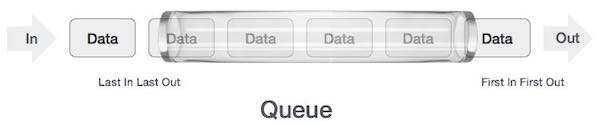top of page
Search

# what is Stack and Queue in C#?## Stack

Stack class represents a last-in, first out collection of object. It is used when you need a last-in, first-out access of items.

• Insertion and deletion happen in a stack from one end only, i.e., the top.

• Insert operation is known as ‘push’ operation.

• Delete operation is known as ‘pop’ operation.

• A pointer is used to access the list, it is known as ‘top’.

• The ‘top’ points to the last element of the list.

• It helps work with problems associated with recursion.

There are three types of public methods in Stack class as described below

1. Count - Gets the number of object contains in stack.

2. Push - Inserts an object at the top of the stack.

3. Pop - Removes and returns the object at the top of stackThe following is the property of Stack class −

• Count− Gets the number of elements in the stack.

The following are the methods of Stack class −

 1 public virtual void Clear();Removes all elements from the Stack. 2 public virtual bool Contains(object obj);Determines whether an element is in the Stack. 3 public virtual object Peek();Returns the object at the top of the Stack without removing it. 4 public virtual object Pop();Removes and returns the object at the top of the Stack. 5 public virtual void Push(object obj);Inserts an object at the top of the Stack. 6 public virtual object[] ToArray();Copies the Stack to a new array.

## Queue

Queue collection class is a concept in C# that is included in the System.Collection namespace. The elements are stored in a QUEUE in FIFO. The first element added will be the first to go out like a queue of people outside a movie hall to buy tickets.

• Insertion and deletion happen from two opposite ends of the list.

• Insertion happens from the rear end.

• Deletion happens from the front end.

• Insert operation is also known as ‘enqueue’.

• Delete operation is also known as ‘dequeue’.

• Two pointers are used to access the list.

• Front pointer points to first element that is inserted in the list, and still present.

• The rear pointer points to the last inserted element of the queue.

• It is used to solve problems that have sequential processing techniques.It has two methods −

1. Enqueue() method to add values

2. Dequeue() method to retrieve values

### 1. Enqueue

`Queue q = new Queue(); q.Enqueue(“Two”); q.Enqueue(“One”);`

### 2. Dequeue

Return items from the queue.

```Queue q = new Queue();
q.Enqueue(“Two”);
q.Enqueue(“One”);

// remove elements
while (q.Count > 0)
Console.WriteLine(q.Dequeue());```

## Example:

The following is an example showing how to work with Stack class and its Push() and Pop() method −

```using System;
using System.Collections;

namespace CollectionsApplication
{
class Program
{
static void Main(string[] args) {
Stack st = new Stack();

st.Push('A');
st.Push('B');
st.Push('C');
st.Push('D');

Console.WriteLine("Current stack: ");
foreach (char c in st) {
Console.Write(c + " ");
}

Console.WriteLine();

st.Push('P');
st.Push('Q');
Console.WriteLine("The next poppable value in stack: {0}", st.Peek());
Console.WriteLine("Current stack: ");

foreach (char c in st) {
Console.Write(c + " ");
}
Console.WriteLine();
Console.WriteLine("Removing values....");
st.Pop();
st.Pop();
st.Pop();
Console.WriteLine("Current stack: ");
foreach (char c in st) {
Console.Write(c + " ");
}
}
}
}```

Source: tutorialpoint.com

Output:

```Current stack:
D C B A
The next poppable value in stack: Q
Current stack:
Q P D C B A
Removing values....
Current stack:
C B A```

## Difference Between Stack and Queue

 Stack Queue A Linear List Which allows insertion or deletion of an element at one end only is called Stack A Linear List Which allows insertion at one end and deletion at another end is called Queue Since insertion and deletion of an element are performed at one end of the stack, the elements can only be removed in the opposite order of insertion. Since the insertion and deletion of an element are performed at the opposite end of the queue, the elements can only be removed in the same order of insertion. A stack is called as Last In First Out (LIFO) List A queue is called First In First Out (FIFO) List. The most and least accessible elements are calledas TOP and BOTTOM of the stack Insertion of the element is performed at the FRONT endand deletion is performed from the REAR end Example: Stack is arranging plates in one above one. Example: Ordinary queue in provisional store. Insertion operation is referred to as PUSH and deletion operation is referred to as POP Insertion operation is referred to as ENQUEUE and deletion operation is referred to as QUEUE Function calling in any languages uses Stack Task Scheduling by Operating System uses a queue To check if a stack is empty, the following condition is used:TOP == -1 To check if a queue is empty, the following condition is used:FRONT == -1 || FRONT == REAR + 1 To check if a stack is full, the following condition is used:TOP == MAX – 1 To check if a queue is full, the following condition is used:REAR == MAX – 1 A Stack requires only one reference pointer. A Queue requires two reference pointers.

The Tech Platform